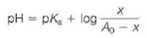# [Solved] In This and Following Chapter's, More Difficult Problems Are Indicated

Question 14
Essay

## In this and following chapter's, more difficult problems are indicated by an asterisk. The efficiency of a buffer in resisting changes in pH upon addition of base or acid is referred to as the buffer capacity. One way to define this quantity, which we shall call B, is B = dx/dp H,where x is the number of moles of base added to a weak acid. Note that the Henderson-Hasselbalch equation may be written as where A 0 is the total concentration of acid. (a) Obtain an expression for B, in terms of A 0 and the fraction of acid titrated f,where f = x /A 0. [Hint: It is easiest to determine dp H/ dx and take the reciprocal] (b) At what value of f is buffer capacity maximal (The easiest way to determine this is to make a graph of B versus f. ) (c) What is the maximal value of B (d) What is the effect of A 0 on B

10+ million students use Quizplus to study and prepare for their homework, quizzes and exams through 20m+ questions in 300k quizzes.

### Biology

Explore our library and get Biochemistry Homework Help with various study sets and a huge amount of quizzes and questions

472

Study sets

13.4K

Quizzes

881.8K

Questions

Upload material to get free accessInvite a friend and get free accessSubscribe and get an instant access